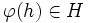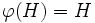Difference between revisions of "Injective endomorphism-invariant subgroup"

BEWARE! This term is nonstandard and is being used locally within the wiki. [SHOW MORE]
This article defines a subgroup property: a property that can be evaluated to true/false given a group and a subgroup thereof, invariant under subgroup equivalence. View a complete list of subgroup properties[SHOW MORE]
If the ambient group is a finite group, this property is equivalent to the property: characteristic subgroup
View other properties finitarily equivalent to characteristic subgroup | View other variations of characteristic subgroup | Read a survey article on varying characteristic subgroup

Definition

QUICK PHRASES: invariant under all injective endomorphisms, injective endomorphism-invariant

Equivalent definitions in tabular format

Below are many equivalent definitions of injective endomorphism-invariant subgroup.

No. Shorthand A subgroup of a group is characteristic in it if... A subgroup$H$ of a group$G$ is called a characteristic subgroup of$G$ if ...
1 injective endomorphism-invariant every injective endomorphism of the whole group takes the subgroup to within itself for every injective endomorphism$\varphi$ of$G$,$\varphi(H) \subseteq H$. More explicitly, for any$h \in H$ and$\varphi \in \operatorname{End}(G)$ that is injective,$\varphi(h) \in H$
2 injective endomorphisms restrict to endomorphisms every injective endomorphism of the group restricts to an endomorphism of the subgroup. for every injective endomorphism$\varphi$ of$G$,$\varphi(H) \subseteq H$ and$\varphi$ restricts to an endomorphism of$H$
3 injective endomorphisms restrict to injective endomorphisms every injective endomorphism of the group restricts to an injective endomorphism of the subgroup for every injective endomorphism$\varphi$ of$G$,$\varphi(H) = H$ and$\varphi$ restricts to an injective endomorphism of$H$
This definition is presented using a tabular format. |View all pages with definitions in tabular format

Formalisms

Function restriction expression

This subgroup property is a function restriction-expressible subgroup property: it can be expressed by means of the function restriction formalism, viz there is a function restriction expression for it.
Find other function restriction-expressible subgroup properties | View the function restriction formalism chart for a graphic placement of this property
Function restriction expression$H$ is an injective endomorphism-invariant subgroup of$G$ if ... This means that injective endomorphism-invariance is ... Additional comments
injective endomorphism$\to$ function every injective endomorphism of$G$ sends every element of$H$ to within$H$ the invariance property for injective endomorphisms
injective endomorphism$\to$ endomorphism every injective endomorphism of$G$ restricts to an endomorphism of$H$ the endo-invariance property for injective endomorphisms; i.e., it is the invariance property for injective endomorphism, which is a property stronger than the property of being an endomorphism
injective endomorphism$\to$ injective endomorphism every injective endomorphism of$G$ restricts to a injective endomorphism of$H$ the balanced subgroup property for injective endomorphisms Hence, it is a t.i. subgroup property, both transitive and identity-true

Relation with other properties

Stronger properties

Property Meaning Proof of implication Proof of strictness (reverse implication failure) Intermediate notions

Weaker properties

Property Meaning Proof of implication Proof of strictness (reverse implication failure) Intermediate notions
characteristic subgroup invariant under all automorphisms injective endomorphism-invariant implies characteristic characteristic not implies injective endomorphism-invariant |FULL LIST, MORE INFO
normal subgroup invariant under all inner automorphisms (via characteristic) (via characteristic) Characteristic subgroup|FULL LIST, MORE INFO

Metaproperties

BEWARE! This section of the article uses terminology local to the wiki, possibly without giving a full explanation of the terminology used (though efforts have been made to clarify terminology as much as possible within the particular context)

Here is a summary:

Metaproperty name Satisfied? Proof Difficulty level (0-5) Statement with symbols
transitive subgroup property Yes injective endomorphism-invariance is transitive If$H \le K \le G$ are groups such that$H$ is injective endomorphism-invariant in$K$ and$K$ is injective endomorphism-invariant in$G$, then$H$ is injective endomorphism-invariant in$G$.
trim subgroup property Yes Obvious reasons 0 In any group$G$, the trivial subgroup$\{ e \}$ and the whole group$G$ are injective endomorphism-invariant in$G$
strongly intersection-closed subgroup property Yes injective endomorphism-invariance is strongly intersection-closed If$H_i, i \in I$, are all injective endomorphism-invariant in$G$, so is the intersection of subgroups$\bigcap_{i \in I} H_i$.
strongly join-closed subgroup property Yes injective endomorphism-invariance is strongly join-closed If$H_i, i \in I$, are all injective endomorphism-invariant in$G$, so is the join of subgroups$\langle H_i \rangle_{i \in I}$